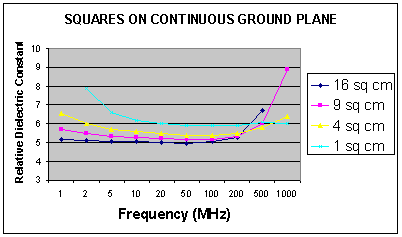# Measuring Relative Dielectric Constant of PCB's Using Parallel Plate Method and a Vector Network Analyser

Introduction

In order to produce PCB transmission lines with a good return loss, we needed a simple and accurate εr measurement method. As an example, to obtain 30 dB return loss requires a track with a characteristic impedance accuracy of better than 3%. This represents a variation of approximately -12.3 % to + 14.7 % in εr for 50 Ohm microstrip on 1.5875 mm thick FR4. When variables such as final exposed track size, etching, and variations in dielectric thickness are taken into account the εr measurement tolerance needs to be much less than this. With this in mind, the following investigation was made and a method evolved.

Aim

To measure the εr of FR4 PCB dielectric to within 1% tolerance using the parallel plate method and a Vector Network Analyser (VNA). To confirm the accuracy of this measurement using a known formula to calculate a 50Ω microstrip, manufacture a sample and measure the return loss.

Method

A series of tests was devised and sample PCB's were manufactured. Three PCB's were etched with four sample areas of 1, 4, 9 and 16 cm2. All sample areas were at least 1 cm from the next nearest sample and from the ground at edge of the board. All measurements were made at the centre of the sample using a semi-rigid cable with the centre conductor passing through the PCB. The phase length of this cable was removed from the test by performing a port extension with the VNA (HP 8753). The boards were tested on a wooden bench and the room was unscreened.

A further PCB with a microstrip was manufactured from the same sheet of material as used for PCB-A to PCB-C (in order to obtain a similar εr). This sample was used to confirm that the resulting return loss was satisfactory.

PCB-A

This has four square samples of 1, 4, 9 and 16 cm2 on the top, and a continuous ground plane on the bottom.

Measurements were made from 1 MHz to 1 GHz and the resultant εr(K) calculated with the following formula.

From Radio Society of Great Britain, Radio Reference Data Book, Sixth Edition p22.

C = 0.0885 KA / d  Formula 1.

Where   C = capacitance in pico Farads

K = dielectric constant

A = area of plate in square centimetres

d = thickness of dielectric in centimetres

From this, we can derive

d = 0.0885 KA / C

The thickness of the dielectric was carefully measured with a micrometer on PCB-B at a point where there was no copper. This measurement is critical and it was found that a vernier caliper does not have the required degree of precision. A number of measurements were made over the exposed dielectric area and a typical measured value was used.

After calculation of the dielectric constant, results of greater than 10 were discarded, because they were obviously wrong and cramped the scale of the valid results. The results of interest are shown below in Figure 1.

Figure 1It can be seen that the measured εrvaries with both frequency and sample size. There is no single point where the curves all coincide. The minimum spread is 11.9% at 200 MHz. Clearly, the measurement accuracy is worse than 1%.

It was decided to change the bottom ground in order to reduce edge capacitance.

1  2  3  4  5  >  Page

Technical Article Index